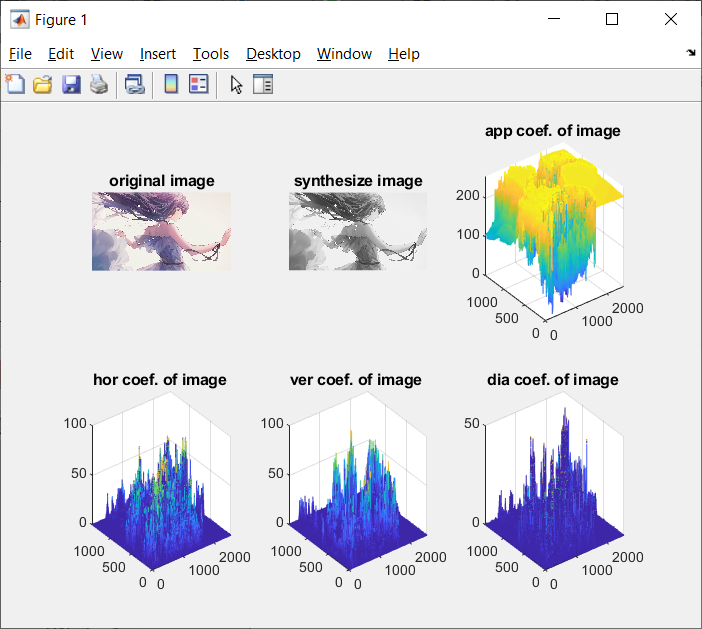Categories

# The MATLAB Image processing and audio signal

Introduction Principles

Discrete Fourier, discrete cosine and discrete wavelet transform is an image, after the audio signal common base operation, a time domain signal is converted to a different transform domain, can lead to different degrees of energy concentration, information hiding use of this principle to select the appropriate location coefficients in the transform domain modification , embedded information, and ensure that the image, audio signal after treatment no significant changes in sensory quality.

Transformation definition

One-dimensional discrete Fourier transform definitions: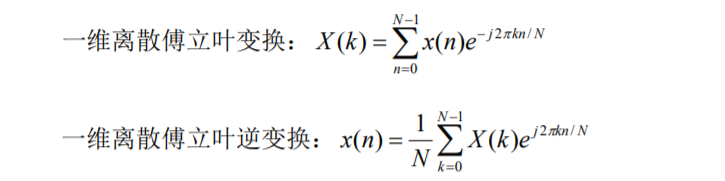One-dimensional discrete cosine transform definition: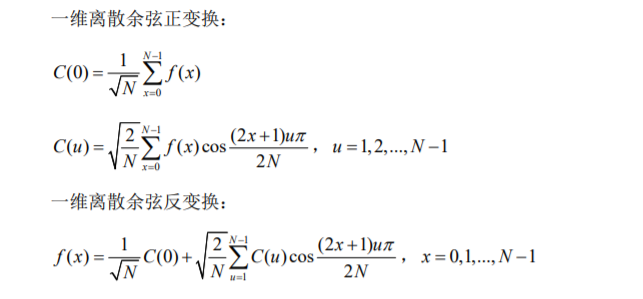A one-dimensional continuous wavelet transform definition: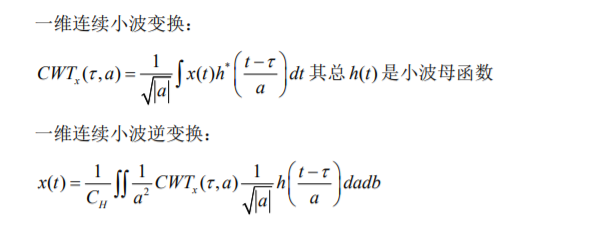Dimensional discrete Fourier transform is defined: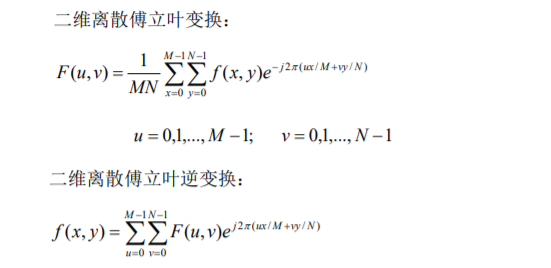Dimensional discrete cosine transform definition: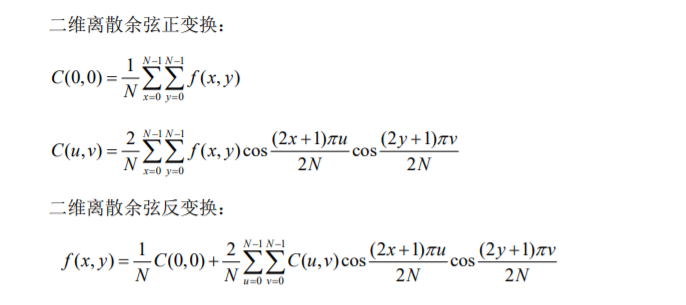Converting the synthesized audio and image analysis with the discrete Fourier

Analysis of synthetic audio file includes the following steps:

l-dimensional discrete Fourier transform

l-dimensional discrete inverse Fourier transform

l observations

The first step: reading the audio file data

M a new file, save as example11.m, enter the following:

clc;

clear;

l = [1, 40000];

[Fn, pn] = uigetfile ( ‘* wav.’, ‘Please select an audio file’);

len = length(x)

uigetfile a file dialog function, provides a graphical interface for the user to select the desired file, return to the target directory name and file name.

Function prototype: y = audioread (FILE)

Function: Reads the audio file format content

Input parameters: file representing audio file name, a string

Return parameters: y means of audio samples, floats

Step two: one-dimensional discrete Fourier transform

M a new file, save as example12.m, enter the following:

```xf = fft(x);

f1 = [0:len-1] * fs / len;

xff = fftshift(xf);

hl = floor(len / 2);

f2 = [-hl:hl] * fs / len;```

fft a function of input parameters and return-dimensional discrete Fourier transform coefficients corresponding to frequencies from 0 to FS (sampling frequency) using a zero frequency corresponding coefficients fftshift moved to the central. The code also calculated frequency value corresponding to the discrete samples in order to better observe the spectrum.

The third step: a one-dimensional inverse discrete Fourier transform

M a new file, save as example13.m, enter the following:

`xsync = ifft(xf);`

ifft function input parameters one-dimensional inverse discrete Fourier transform and returns the coefficients.

Step Four: Observations

M a new file, save as example14.m, enter the following:

```figure;

subplot(2, 2, 1);plot(x);title('original audio');

subplot(2, 2, 2);plot(xsync);title('synthesize audio');

subplot(2, 2, 3);plot(f1, abs(xf));title('fft coef. of audio');subplot(2, 2, 4);plot(f2(1:len), abs(xff));title('fftshift coef. of auio');```

figure (n) represents the creation of the n-th graphics window.

subplot sub drawing function, a first, two parameters specify a sub-image layout, for example, if the parameter is 2, 3 is a screen divided into two rows, each row has three sub-images. The third parameter indicates the number sub-images, the sort order from left to right, top to bottom.

plot is a plot function, default mode of plot (y), y is the parameter data to be drawn; if the horizontal axis shows the need to specify the image sequence, the command line plot (X, y), the default mode is equivalent to plot ([0. .len-1], y), len is the length of the sequence y.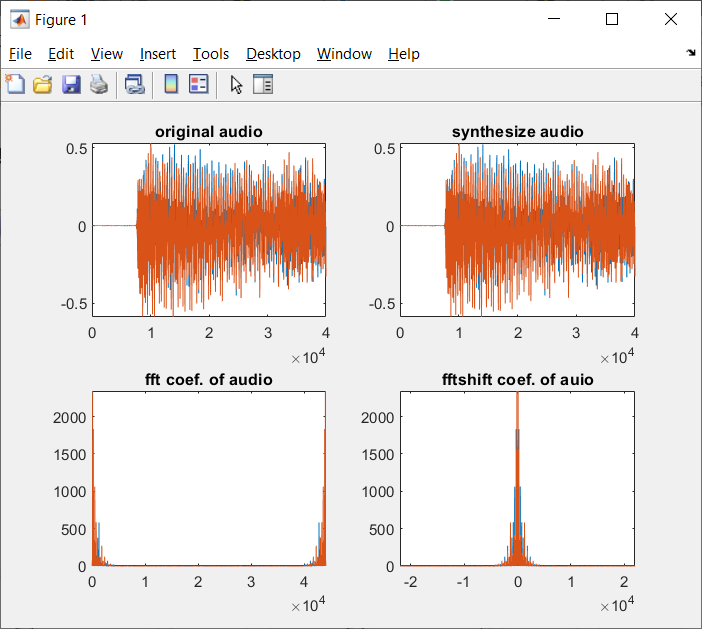Analysis of the composite image file comprising the steps of:

l-dimensional discrete Fourier transform

l two-dimensional inverse discrete Fourier transform

l observations

The first step: reading the image file data

M a new file, save as example21.m, enter the following:

```[fn, pn] = uigetfile('*.png', '请选择图像文件');

[x, map] = imread(strcat(pn, fn), 'png');

I = rgb2gray(x);```

Function Prototype: A = imread (filename, fmt)

Function: Reads fmt specifies the format of the image file contents

Input parameters: filename represents the image file name, image file format string Fmt indicate name, string functions supported image formats include: JPEG, TIFF, GIF, BMP, etc., when the parameter is not included in the file name format, the function attempts inferred file format.

Return parameter: A represents the content of the image data, the integer rgb2gray RGB image is converted into grayscale.

Step two: two-dimensional discrete Fourier transform

M a new file, save as example22.m, enter the following:

```xf = fft2(I);

xff = fftshift(xf);```

fft2 function input parameters and returns a two-dimensional discrete Fourier transform coefficients, using the zero frequency corresponding coefficients fftshift moved to the central.

The third step: two-dimensional inverse discrete Fourier transform

M a new file, save as example23.m, enter the following:

`xsync = ifft2(xf);`

ifft2 function input parameters and two-dimensional discrete Fourier inverse transform which returns coefficient.

Step Four: Observations

M a new file, save as example24.m, enter the following:

```figure;

subplot(2, 2, 1);imshow(x);title('original image');

subplot(2, 2, 2);imshow(uint8(abs(xsync)));title('synthesize image');

subplot(2, 2, 3);mesh(abs(xf));title('fft coef. of image');

subplot(2, 2, 4);mesh(abs(xff));title('fftshift coef. of image');```

imshow mapping function is two-dimensional data, mesh data displayed by the three-dimensional plane.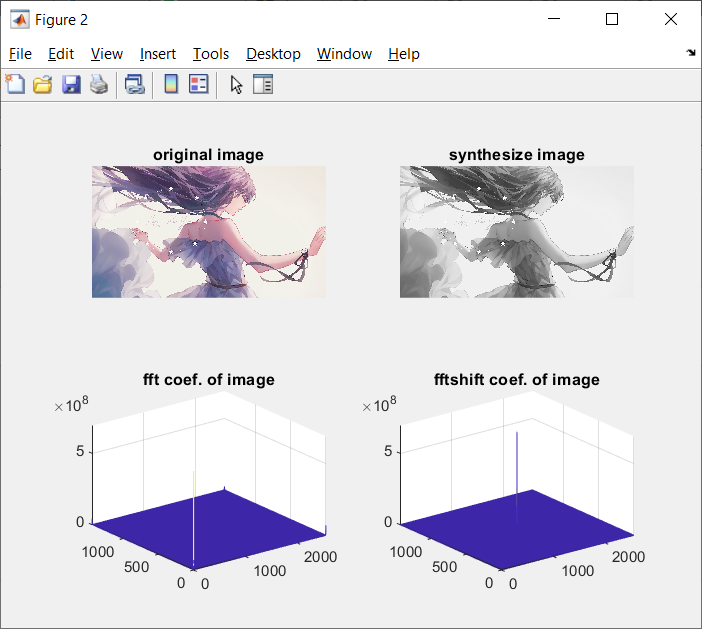Audio and image analysis by synthesis transform using a discrete cosine

Analysis of synthetic audio file includes the following steps:

l one-dimensional discrete cosine transform

l one-dimensional Inverse Discrete Cosine Transform

l observations

The first step: a one-dimensional discrete cosine transform

M a new file, save as example31.m, enter the following:

`xf = dct(x);`

dct a function of the input parameters and returns dimensional Discrete Cosine Transform coefficients, corresponding to frequencies from 0 to FS (sampling frequency).

Step two: one-dimensional Inverse Discrete Cosine Transform

M a new file, save as example32.m, enter the following:

```xsync = idct(xf);

[row,col]=size(x);

xff=zeros(row,col);

xff(1:row,1:col)=xf(1:row,1:col);

y=idct(xff);```

idct function input parameters one-dimensional inverse discrete cosine transform and returns the coefficients. Commonly used in the discrete cosine transform image compression, may attempt to use only part of the reconstructed coefficients language, can be found by observation, the original audio of both audio and synthetic little difference.

The third step: Observations

M a new file, save as example33.m, enter the following:

```figure;

subplot(2, 2, 1);plot(x);title('original audio');

subplot(2, 2, 2);plot(xsync);title('synthesize audio');

subplot(2, 2, 3);plot(f1, abs(xf));title('fft coef. of audio');

subplot(2, 2, 4);plot(f2(1:len), abs(xff));title('fftshift coef. of auio');```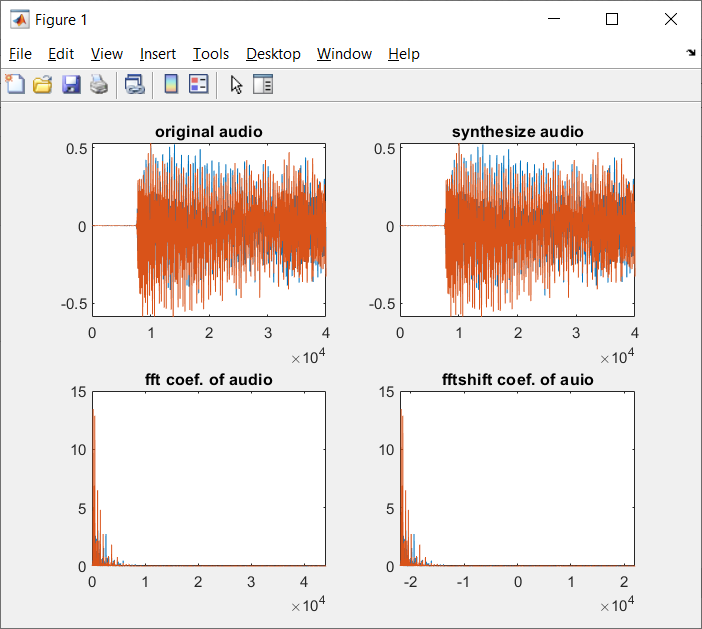Analysis of the composite image file comprising the steps of:

l dimensional discrete cosine transform

l two-dimensional Inverse Discrete Cosine Transform

l observations

The first step: two-dimensional discrete cosine transform

M a new file, save as example41.m, enter the following:

`xf = dct2(I);`

dct2 function input parameters and returns dimensional discrete cosine transform coefficients.

Step two: two-dimensional Inverse Discrete Cosine Transform

M a new file, save as example42.m, enter the following:

```xsync = uint8(idct2(xf));

[row, col] = size(I);

lenr = round(row * 4 / 5);

lenc = round(col * 4 / 5);

xff = zeros(row, col);

xff(1:lenr, 1:lenc) = xf(1:lenr, 1:lenc);

y = uint8(idct2(xff));```

idct2 function input parameters a two-dimensional inverse discrete cosine transform and returns the coefficients. Can try to use a part of coefficients reconstructed image, the present embodiment uses the coefficient matrix data 4/5, other portions of zero. In order to ensure the proper image can be displayed using the reconstructed image uint8 original data type conversion data, to ensure that their value range is between 0 and 255.

The third step: Observations

Please enter a command displayed four sub FIG, respectively, is an original image, using all the coefficients restored image, using the image display portion and coefficients restored three-dimensional perspective view of coefficients.

M a new file, save as example43.m, enter the following:

```figure;

subplot(2, 2, 1);imshow(x);title('original image');

subplot(2, 2, 2);imshow(uint8(abs(xsync)));title('synthesize image');

subplot(2, 2, 3);imshow(uint8(abs(y)));title('part synthesize image');

subplot(2, 2, 4);mesh(abs(xff));title('fftshift coef. of image');```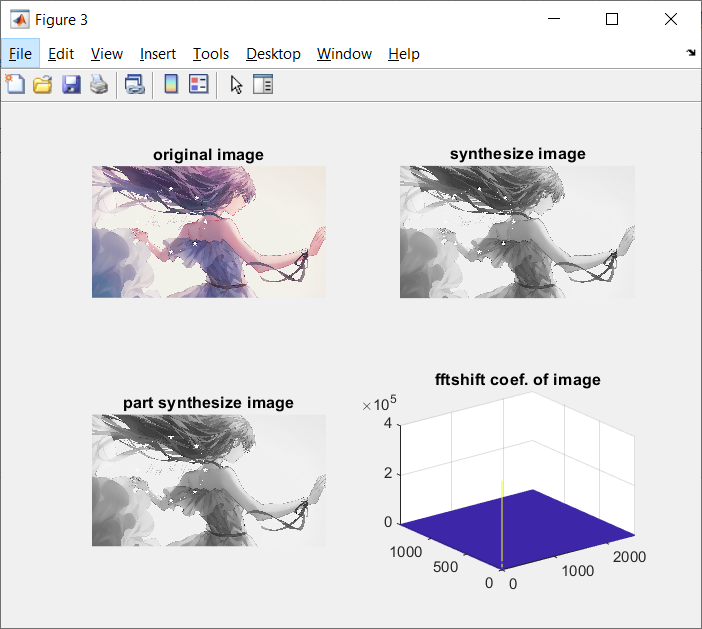Synthesis of audio and image analysis using discrete wavelet transform

Analysis of synthetic audio file includes the following steps:

l-dimensional discrete wavelet transform

l one-dimensional inverse discrete wavelet transform

l observations

The detailed operation steps:

The first step: a one-dimensional discrete wavelet transform

M a new file, save as example51.m, enter the following:

`[C, L] = wavedec(x, 2, 'db4');`

wavedec function of the input parameters and the one-dimensional discrete wavelet transform coefficients and coefficient C is returned to its length L. levels The second argument specifies the number of stages of the wavelet transform, the third argument specifies the name of Wavelet Transform Wavelet used.

Step two: one-dimensional inverse discrete wavelet transform

M a new file, save as example52.m, enter the following:

```xsync = waverec(C, L, 'db4');

cA2 = appcoef(C, L, 'db4', 2);

cD2 = detcoef(C, L, 2);

cD1 = detcoef(C, L, 1);```

waverec a function of input parameters dimensional inverse discrete wavelet transform and returns the coefficients.

appcoef wavelet coefficient approximation component returns, the first parameter C, the second parameter is wavedec return parameter L, and all levels of the wavelet coefficients of its length, the third argument specifies the name of the wavelet basis, the fourth parameter specified level.

Back wavelet coefficients detcoef detail component, the first parameter C, the second parameter is wavedec return parameter L, and all levels of the wavelet coefficients of its length, the third argument specifies the number of stages.

The third step: Observations

M a new file, save as example53.m, enter the following:

```figure;

subplot(2, 3, 1);plot(x);title('original audio');

subplot(2, 3, 2);plot(xsync);title('synthesize audio');

subplot(2, 3, 4);plot(cA2);title('app coef. of audio');

subplot(2, 3, 5);plot(cD2);title('det coef. of auio');

subplot(2, 3, 6);plot(cD1);title('det coef. of auio');```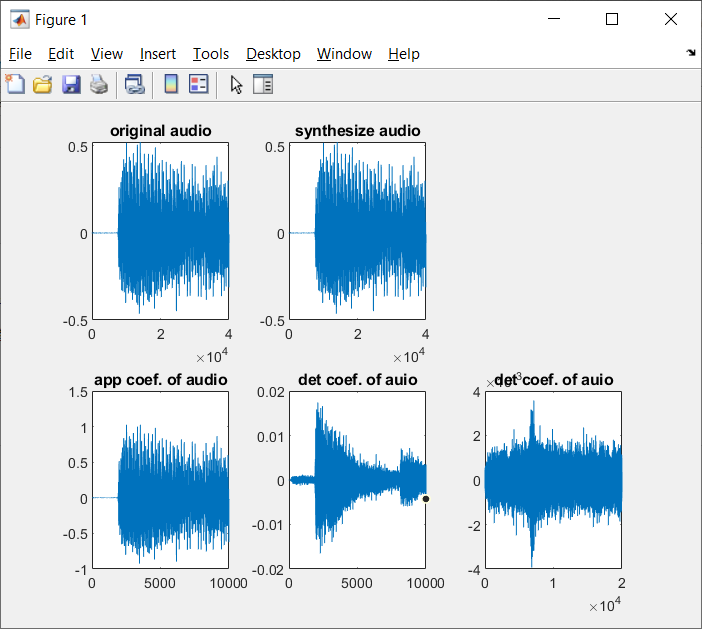Analysis of the composite image file comprising the steps of:

l dimensional discrete wavelet transform

l-dimensional inverse discrete wavelet transform

l observations

The first step: two-dimensional discrete wavelet transform

M a new file, save as example61.m, enter the following:

```sx = size(I);

[cA1, cH1, cV1, cD1] = dwt2(I, 'bior3.7');```

dwt2 function input parameters and two-dimensional discrete wavelet transform returns a component approximately horizontal detail component, the vertical detail component and diagonal detail component.

If the image is to be multi-level wavelet decomposition, use wavedec2 function.

Step two: two-dimensional inverse discrete wavelet transform

M a new file, save as example62.m, enter the following:

```xsync = uint8(idwt2(cA1, cH1, cV1, cD1, 'bior3.7', sx));

A1 = uint8(idwt2(cA1, [], [], [], 'bior3.7', sx));

H1 = uint8(idwt2([], cH1, [], [], 'bior3.7', sx));

V1 = uint8(idwt2([], [], cV1, [], 'bior3.7', sx));

D1 = uint8(idwt2([], [], [], cD1, 'bior3.7', sx));```

idwt2 function dimensional discrete input parameters and returns the inverse wavelet transform coefficients. You can try to use only a component approximately horizontal detail component, the vertical detail component or diagonal detail component of the reconstructed image.

The third step: Observations

FIG six sub display command input, respectively, of the original image, using an image restoration all coefficients, wavelet coefficients approximation component, the level of detail components, the vertical detail component and diagonal detail component.

M a new file, save as example63.m, enter the following:

```figure;

subplot(2, 3, 1);imshow(x);title('original image');

subplot(2, 3, 2);imshow(uint8(abs(xsync)));title('synthesize image');

subplot(2, 3, 3);mesh(A1);title('app coef. of image ');

subplot(2, 3, 4);mesh(H1);title('hor coef. of image ');

subplot(2, 3, 5);mesh(V1);title('ver coef. of image ');

subplot(2, 3, 6);mesh(D1);title('dia coef. of image ');```